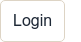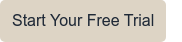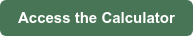# How to Calculate Delivery Cost for Your FarmDelivery costs can be very expensive and hard to track. When first starting to offer delivery in your business, it is important to calculate the costs of being on the road to ensure it’s profitable for you to offer that service to your customers.

Want to skip to calculating hourly distribution costs, your break-even point, target profit and more? Check out our Free Calculators, otherwise, read on below for 6 helpful steps ⬇️

## Step 1: Determine time on the road

The first step is to calculate how many hours the vehicle is on the road on a daily basis.

For example, on any given delivery day, the truck might be on the road for 6 hours each day and deliveries are made on Mondays, Thursdays and Saturdays (three times a week).

6 hours/day x 3 days = 18 hours per week

## Step 2: Cost of driver

This is dependent on the cost of your specific driver, whether that is you personally or a third party.

For this example, let’s say the cost of your driver is \$30/hour.

\$30/hour x 6 hours/day x 3 days = \$540/week

## Step 3: Cost of gas

Cost of gas can be a tricky variable to determine. The easiest way to determine the cost of gas is to fill up the transportation vehicle fully with gas at the beginning of a delivery day, note the cost, and at the end of the day calculate the cost of the gas used for that day.

For example, let's say the cost to fill up the vehicle completely was \$100. At the end of the day, ¾ of the tank has been used; therefore the cost of the fuel used was \$75 (\$100 x 75% = \$75). The vehicle was on the road for 6 hours. The cost of gas per hour would be \$12.50 (\$75/6 hours = \$12.50).

Note: this is an approximation of the cost of gas and may vary based on traffic or route changes.

## Step 4: Determine hourly insurance cost

What is your annual insurance cost? Take that number and divide by 12 months to determine monthly cost. To determine hourly cost, divide the cost of insurance monthly by the number of hours driven in a month.

For example, let’s say the annual insurance cost is \$3,000.

The monthly insurance cost would be \$250 (\$3,000/12 months = \$250).

The number of hours driven in a month for this vehicle is 72 hours (3 days/week x 4 weeks = 12 days a month; 12 days x 6 hours = 72 hours/month).

The hourly rate for insurance would be \$3.47 (\$250/month / 72 hours/month = \$3.47).

## Step 5: Determine hourly cost of depreciation

Determine the percent of depreciation per year for your vehicle. We recommend applying at least a 15% depreciation on the value of a vehicle per year, however it can be dependent on each case.

To determine annual depreciation value, multiply the value of your vehicle if you were to sell it today by the annual depreciation percentage. To determine monthly depreciation divide the annual depreciation by 12 months. To determine hourly depreciation, divide the monthly depreciation by the number of hours driven each month.

For our example, the value of our vehicle today is \$50,000.

With an annual depreciation of %15, the annual depreciation value would be \$7,500. (\$50,000 x 15% = \$7,500).

The hourly depreciation would on the vehicle would be \$8.68. (\$7,500/12 months = \$625; \$625/72 hrs = \$8.68).

## Step 6: Total hourly delivery costs

The final step to calculate hourly delivery costs is to sum every step. The hourly delivery cost for our example would be \$54.65.

(\$30 for cost of driver + \$12.50 for cost of gas + \$3.47 for cost of insurance + \$8.68 for cost of depreciation = \$54.65/hour)

### Optimize profitability on all of your delivery orders

Download our Minimum Order Calculator to calculate hourly distribution costs, your break-even point, target profit and more.